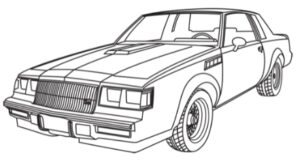# Decoding the Buick Grand National VIN Number

Example for a 1984 Regal Grand National: 1G4AK479XEH400001:

1 = Manufacturing Country (USA)
G = Manufacturer (GM)
4 = Division (1 = Chevy, 2 = Pontiac, 3 = Oldsmobile, 4 = Buick, 5 = GMC, 6 = Cadillac)
A = Restraint System
K = Sales/Option leve (Regal T-Type)
4 = Body Code (2 Door Coupe)
7 = Body Code (2nd digit)
9 = Engine Code (LM9 3.8L SFI Turbo)
X – Check Digit
E = 1985 Model year (E = 1984)
H = Plant Code (Flint Michigan)
400001 = Serial number

Example for a 1985 Regal Grand National: 1G4GK479XFP400001:

1 = Manufacturing Country (USA)
G = Manufacturer (GM)
4 = Division (1 = Chevy, 2 = Pontiac, 3 = Oldsmobile, 4 = Buick, 5 = GMC, 6 = Cadillac)
G = Series (G body)
K = Sales/Option level (Regal T-Type)
4 = Body Code (2 Door Coupe)
7 = Second Digit
9 = Engine Code (LM9 3.8L SFI Turbo)
X – Check Digit
F = Model year (F = 1985)
P = Plant Code (Pontiac Michigan)
400001 = Serial number

Example for a 1986 Regal Grand National:  1G4GK477XGP400001:

1 = Manufacturing Country (USA)
G = Manufacturer (GM)
4 = Division (1 = Chevy, 2 = Pontiac, 3 = Oldsmobile, 4 = Buick, 5 = GMC, 6 = Cadillac)
G = Series (G body)
K = Sales/Option level (Regal T-Type)
4 = Body Code (2 Door Coupe)
7 = Second Digit
7 = Engine Code (LC2 3.8L SFI Turbo)
X – Check Digit
G = Model year (G = 1986)
P = Plant Code (Pontiac Michigan)
400001 = Serial number

Example for a 1987 Regal Grand National: 1G4GJ117XHP400001:

1 = Manufacturing Country (USA)
G = Manufacturer (GM)
4 = Division (1 = Chevy, 2 = Pontiac, 3 = Oldsmobile, 4 = Buick, 5 = GMC, 6 = Cadillac)
G = Series (G body)
J = Sales/Option level (Regal, the letter K was supposed to be for a Grand National, but wasn’t used)
1 = Body Code (2 Door Coupe)
1 = Restraint System (manual belts)
7 = Engine Code (LC2 3.8L SFI Turbo)
X – Check Digit
H = Model year (H = 1987)
P = Plant Code (Pontiac Michigan)
400001 = Serial number

.

## Related postsHey, Turbo Buick Fan!